# How to Use TIME Function in Excel (8 Suitable Examples)

Last updated: September 21, 2023

The TIME function is a built-in function in Excel that is categorized as a Date/Time Function. TIME function of Microsoft Excel is one of the essential functions. We use this in a lot of scenarios. Besides, you can perform various calculations with this. For that reason, it will save you a lot of time. In this tutorial, you will learn every detail of the TIME function in Excel. This tutorial will be on point with suitable examples and proper illustrations.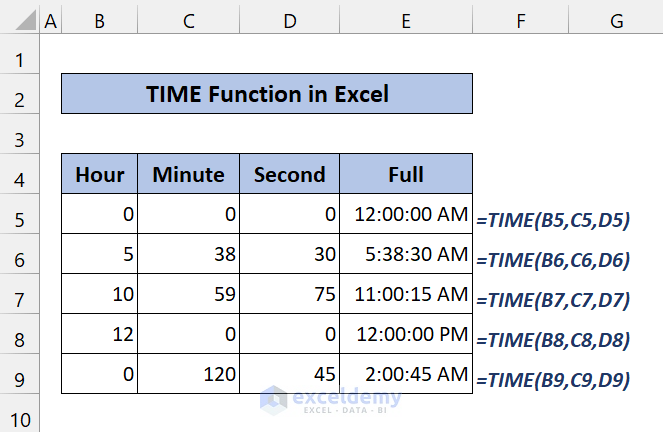The above screenshot is an overview of the article which represents a simplified application of the TIME function in Excel. You’ll learn more about the dataset as well as the methods and functions under different criteria in the following sections of this article.

## Introduction to the TIME Function

Function Objective

Returns the decimal number for an individual time. If the cell format was General before the function was given, it will format the result as a date.

It returns a decimal number that varies from 0 to 0.99988426. 0 represents the time 0:00:00 (12:00:00 AM) and   0.99988426 represents the time 23:59:59 (11:59:59 PM).

Syntax

[wpsm_box type=”solid_border” float=”none” textalign=”center”]
=TIME(hour, minute, second)
[/wpsm_box]

Arguments Explanations

[wpsm_colortable color=”black”]

Argument Required/Optional Explanations
hour Required A number from 0 (zero) to 32767 represents the hour. Any value greater than 23 will be divided by 24 and the remainder will be treated as the hour value. For example, TIME(27,0,0) = TIME(3,0,0) = .125 or 3:00 AM.
minute Required A number from 0 to 32767 representing the minute. Any value greater than 59 will be converted to hours and minutes. For example, TIME(0,750,0) = TIME(12,30,0) = .520833 or 12:30 PM.
second Required A number from 0 to 32767 representing the second. Any value greater than 59 will be converted to hours, minutes, and seconds. For example, TIME(0,0,2000) = TIME(0,33,22) = .023148 or 12:33:20 AM.

[/wpsm_colortable]

Returns

It returns a numeric value between 0 and 0.999988426.

Available in

Excel for Office 365, Excel 2019, Excel 2016, Excel 2013, Excel 2011 for Mac, Excel 2010, Excel 2007, Excel 2003, Excel XP, Excel 2000

## How to Use TIME Function in Excel

When you use the TIME function, it will give you a decimal value. If you don’t want that, you have to change it from the Number Format.

Take a look at the following example: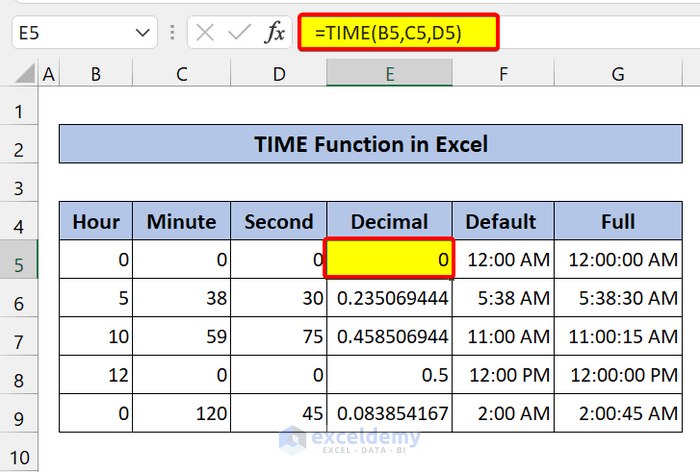Here, you can see, we have some decimal numbers for the Hour, Minute, and Second. After that, it gave us a numeric value for that time. It happened because we set the format as General.

In the next columns, you can see, it shows the default format and the full format of time. Now, how do we do that?

We just change the format from General to Time. Click on any decimal number in the column. From the Home tab, you can go to the Number group to change the format.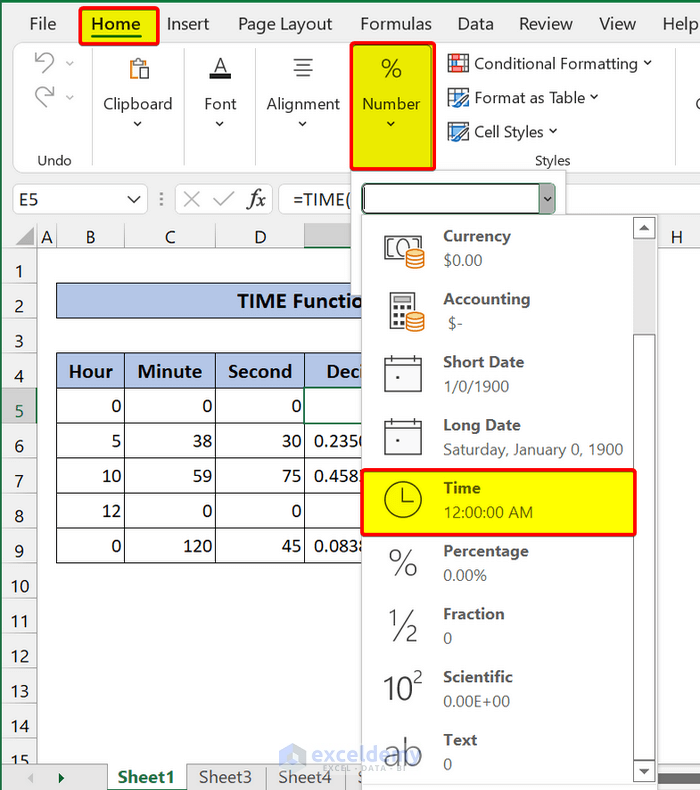Another way you can do this is, press Ctrl+1 on your keyboard. Then the Format cells dialog box will appear. Select Time from there. Then choose any suitable time format you want.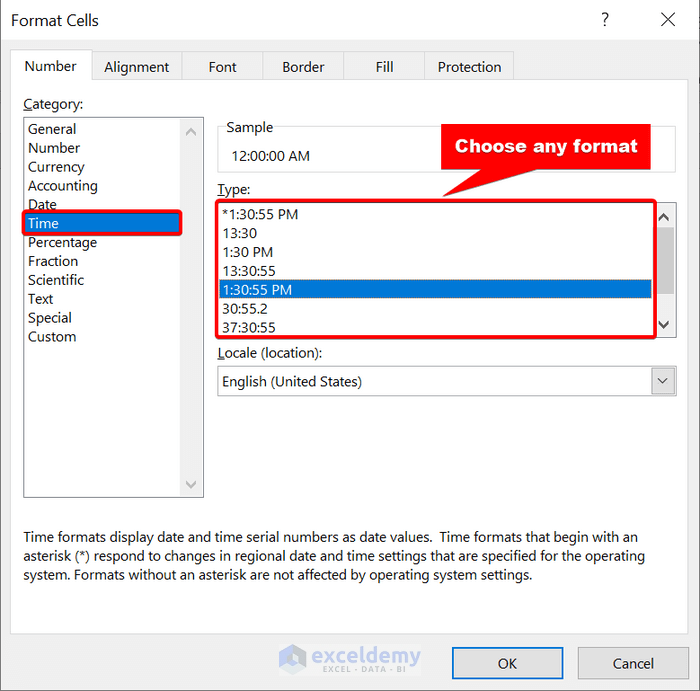## 8 Useful Examples of TIME Function in Excel

In the following section, we are providing you with seven suitable and effective examples that you can implement into your dataset. Make sure you check them all. We recommend you learn and apply all these. It will surely enrich your Excel knowledge.

### 1. Some Basic Uses of TIME Function

In this section, we are just clearing some basic concepts. Look at these following codes:

[wpsm_box type=”gray” float=”none” textalign=”center”]

`=TIME(7,0,0) // 7 hours`

`=TIME(0,10,0) // 10 minutes`

`=TIME(0,0,35) // 35 seconds`

`=TIME(2,30,0) // 2.5 hours`

[/wpsm_box]

When you input the numbers as 7 hours or 10 minutes. It will add the hours with the time 12:00:00 AM.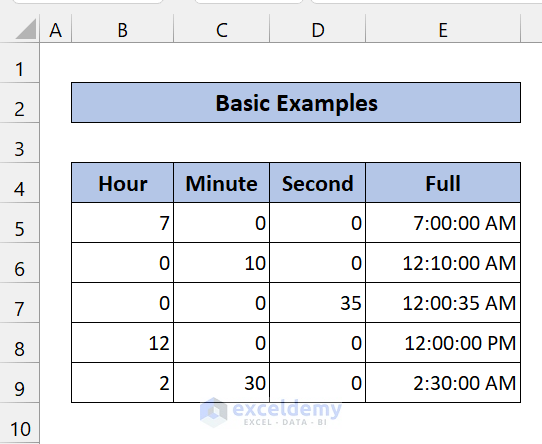The TIME function decode units in a bigger form. For example, if you input these in the TIME function, both of them will add 4 hours to 12:00:00 AM.

[wpsm_box type=”gray” float=”none” textalign=”center”]

`=TIME(12,0,0) // 12 hours`

`=TIME(36) // 12 hours`

[/wpsm_box]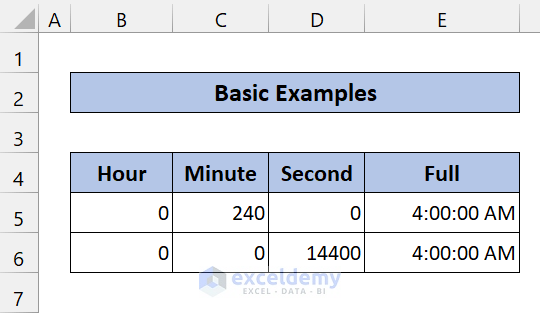If your total time crosses 24, it will reset the time to 12:00:00 AM.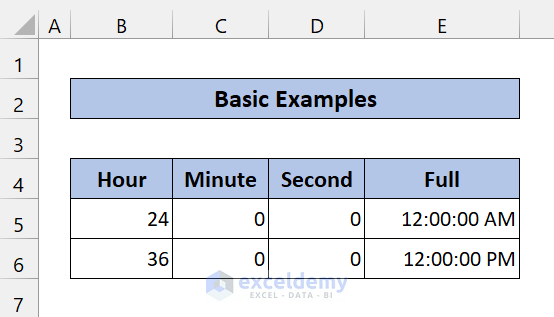### 2. Adding Time Using Excel TIME Function

You can normally add two times with the TIME function in Excel. It is normal to add two numbers. This is pretty easy to perform.

Type the following formula in Cell C8:

[wpsm_box type=”gray” float=”none” textalign=”center”]
`=TIME(B5,C5,D5)+TIME(B6,C6,D6)`
[/wpsm_box]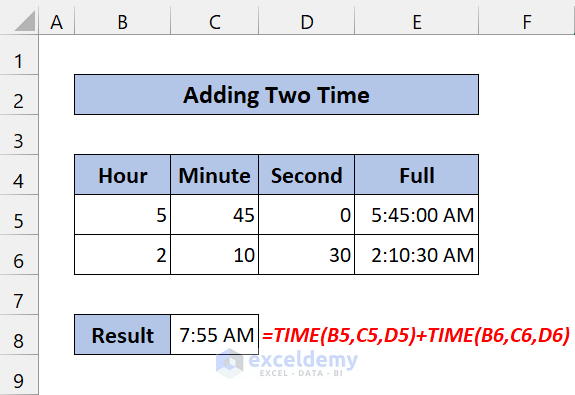As you can see, we have successfully added two times.

If you have two times in full format, you can also add them with the TIME function. We are performing that with the combination of the HOUR function, the MINUTE function, and the SECOND function.

Take a look at the following screenshot to have a better understanding: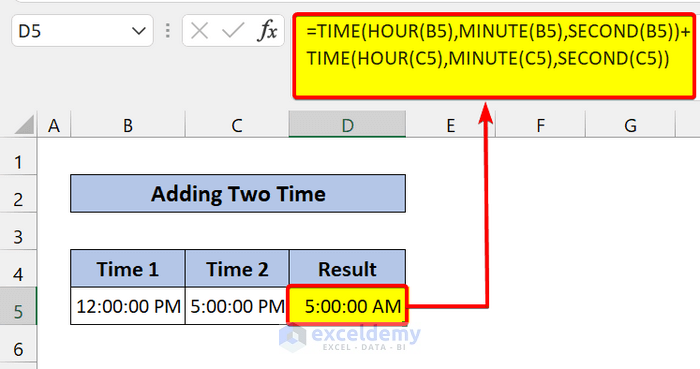### 3. Subtracting One Time from Another in Excel

Similar to the previous example, you can also subtract one time from another. This is also the same as subtracting two numbers.

Type the following formula in Cell C8:

[wpsm_box type=”gray” float=”none” textalign=”center”]
`=TIME(B5,C5,D5)-TIME(B6,C6,D6)`
[/wpsm_box]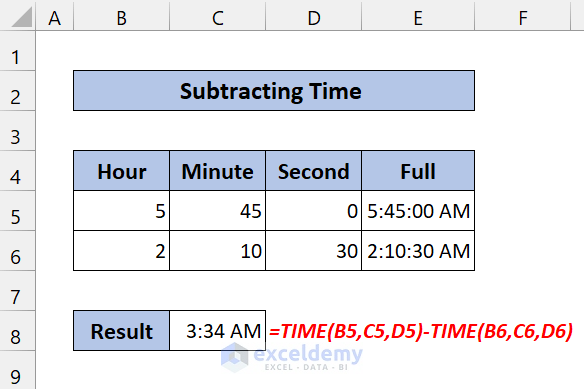As you can see, we have successfully subtracted two times.

If you have two times in full format, you can also subtract them with the TIME function. We are performing that with the combination of the HOUR function, the MINUTE function, and the SECOND function.

Take a look at the following screenshot to have a better understanding: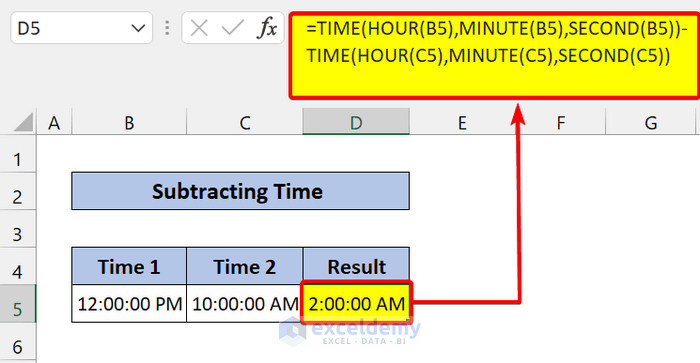### 4. Calculate Elapsed Time Using TIME Function

If you want to know, how much time has elapsed since the start time to now, you can simply use the NOW function. It will return the current time, and then subtract the time from it.

Type the following formula in Cell E5:

[wpsm_box type=”gray” float=”none” textalign=”center”]
`=NOW()-TIME(B5,C5,D5)`
[/wpsm_box]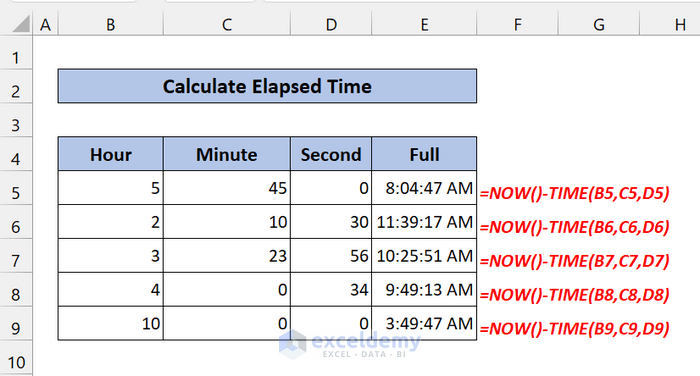As you can see, we have successfully used the TIME function to find the elapsed time in Excel.

### 5. Add or Subtract Hours to Time in Excel

Now, you can add hours in a given time. You can perform this by using the TIME function. If you want to find out the time after a particular hour passes, you can use this method.

Type the following formula in Cell D5 and drag the fill handle icon:

[wpsm_box type=”gray” float=”none” textalign=”center”]
`=B5+TIME(C5,0,0)`
[/wpsm_box]

You can understand this from the following screenshot: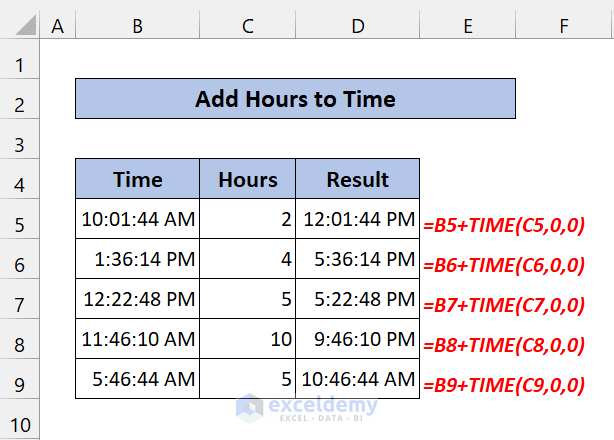You can perform the subtraction in a similar fashion.### 6. Add or Subtract Minutes to Time in Excel

Similar to the previous method, you can also add minutes to any time. Just type the following formula in Cell D5:

[wpsm_box type=”gray” float=”none” textalign=”center”]
`=B5+TIME(0,C5,0)`
[/wpsm_box]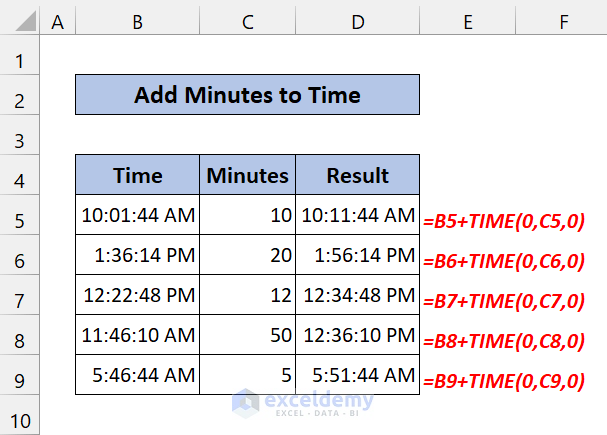As you can see, we have successfully added minutes to time using the TIME function in Excel.

You can change the plus sign to minus to subtract minutes.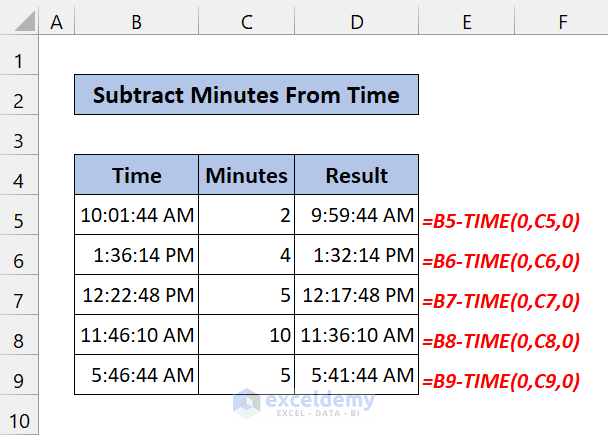### 7. Add or Subtract Seconds to Time in Excel

If you read the previous section, I think you already know the formula. You have to just change the cell reference to second in TIME function.

Formula we are using :

[wpsm_box type=”gray” float=”none” textalign=”center”]
`=B5+TIME(0,0,C5)`
[/wpsm_box]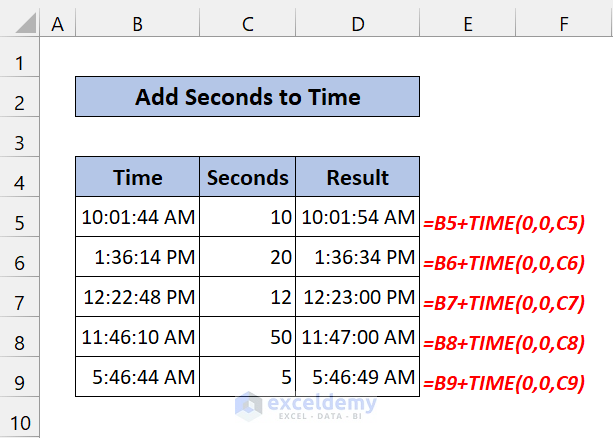Similarly, you can add hours, minutes, and seconds together in one TIME function.

Do the subtraction in a similar way.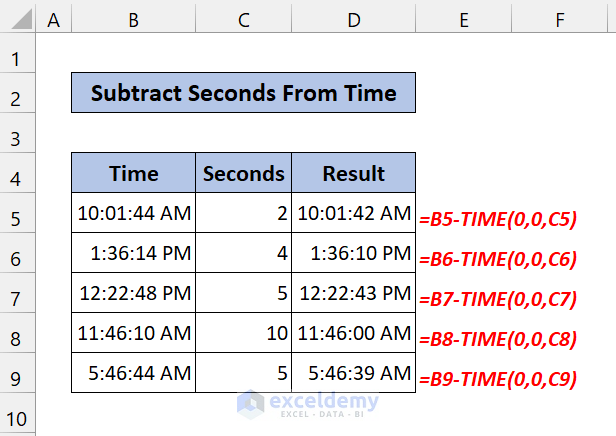### 8. Adding Multiple Times in Excel

If you have multiple time values in a dataset, you find the total sum of those. You have to use the SUM function to add them.  Suppose, you have a few assignment times in column C and you want to add them up. Just type the SUM formula like `=SUM(C5:C9) `and get the result in the default format, like hh:mm:ss.

Take look at the following screenshot to have a better understanding: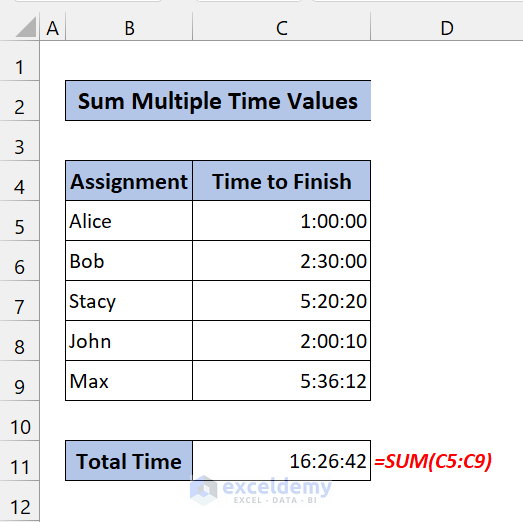## 💬 Things to Remember

If the total time reaches 24 hours, it will reset to zero (12:00:00 AM)

If the given hours are less than zero, it will show #NUM! Error or ####.

If the arguments are non-numeric, it will show #VALUE! error.

Uses of TIME Function.xlsx
[/wpsm_box]

## Conclusion

To conclude, I hope this tutorial has provided you with a piece of useful knowledge to use the TIME function in Excel. We recommend you learn and apply all these instructions to your dataset. Download the practice workbook and try these yourself. Also, feel free to give feedback in the comment section. Your valuable feedback keeps us motivated to create tutorials like this.

Keep learning new methods and keep growing!

## Related ArticlesA.N.M. Mohaimen Shanto

Hello! I am Shanto. An Excel & VBA Content Developer. My goal is to provide our readers with great tutorials on various Excel-related problems. I hope our easy but effective tutorials will enrich your knowledge. I have completed my BSc in Computer Science & Engineering from Daffodil International University. Working with data was always my passion. Love to work with data, analyze those, and find patterns. Also, love to research. Always look for challenges to keep me growing.

We will be happy to hear your thoughts